# College Physics : Kinetic Energy

## Example Questions

### Example Question #1 : Kinetic Energy

A car traveling at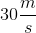has a kinetic energy. If the car accelerates to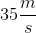, what will the new kinetic energy be?

The mass of the car is required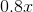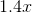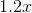Explanation:

Kinetic energy is given by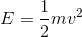. We will begin by calculating the car's initial kinetic energy, in terms of the unknown mass of the car: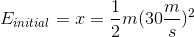.

Next, we will calculate the final energy of the car, also in terms of the unknown mass of the car: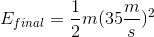.

To find the ratio of the final to initial kinetic energy, we divide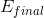by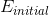. We see that this reduces to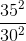with the both the massandterms cancelling.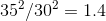. Thus, the new kinetic energy is.

### Example Question #1 : Mechanics

A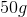steel ball is in a spring loaded launcher. The spring has a constant of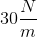. If the ball is pulled back 50 cm then released and the ball leaves the launcher at a speed of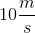, how much work was done by friction?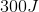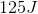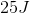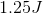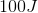Explanation:

So in order to solve this problem, we first should think about where all the energy in the system is coming from and going. Specifically when you pull the spring back, the work you do is turned into potential energy stored in the spring. So: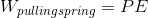and the energy transforms into kinetic when you let go, but some of that energy is lost to friction so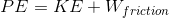and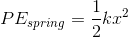So use the equation below, enter in your known quantities and then solve for the work done by friction.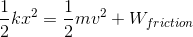Plug in known values and solve.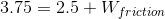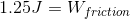### All College Physics Resources